Refer to our Texas Go Math Grade 5 Answer Key Pdf to score good marks in the exams. Test yourself by practicing the problems from Texas Go Math Grade 5 Lesson 8.2 Answer Key Addition and Subtraction Equations.

Unlock the Problem

Yara is going camping in the Everglades National Park. Her backpack with camping gear weighs 17 pounds. When she adds her camera gear, the total weight of her backpack is 25 pounds. How much does Yara’s camera gear weigh?
• What does the backpack weigh without the camera gear?
________________
• What do you need to find?
_________________
The backpack weigh without the camera gear is 17 pounds.
We need to find out the camera gear weigh.

Write a related equation.
The problem describes a part-part-whole relationship. A strip diagram can help you understand this relationship and write an equation. Let c represent the weight (in pounds) of the camera gear.STEP 1: Use the strip diagram to write an equation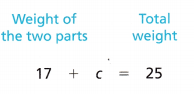STEP 2: Write a subtraction equation that is related to the addition equation.
Equation: _______________
Subtract: c = _______________
So, Yara’s camera gear weighs _______________ pounds.
Equation: 17 + c = 25
Subtract: c = 25 – 17 = 8
So, Yara’s camera gear weighs 8 pounds.

What if Yara’s backpack with camping gear weighs 15 pounds and the camera gear weighs 6 pounds? How much does her backpack with camping gear and camera gear weigh? How would the strip diagram above change?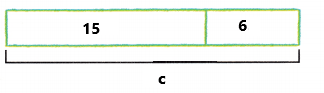15 pounds + 6 pounds = 21 pounds
Yara’s backpack with camping gear and camera gear weigh is 21 pounds.
Explanation:
Yara’s backpack with camping gear weighs 15 pounds and the camera gear weighs 6 pounds. Add 15 pounds with 6 pounds the sum is 21 pounds. Yara’s backpack with camping gear and camera gear weigh is 21 pounds.

Math Talk
Mathematical Processes

Explain how you can tell that your solution to an equation is true.

Example

Use a related equation.
Kent has a collection of CDs. He gives 5 CDs to his brother. He then has 8 CDs left in his collection. How many CDs did Kent have before he gave some to his brother?
• How many CDs did Kent give away?
_________________
• What operation will you use to show the CDs that Kent gave away?
___________________
Kent gave 5 CDs to his brother.
I will use subtraction operation to show the CDs that Kent gave away.

STEP 1: Draw a strip diagram.
• Write the missing labels in the strip diagram, where c is the number of CDs in Kent’s collection before he gave some away.
• Use the model to write the equation.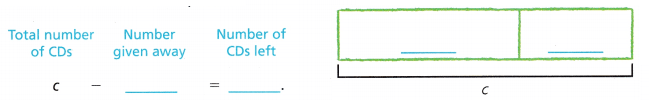STEP 2: Use the strip diagram to write a related addition equation. Then add to solve.
Equation: _______________
So, Kent had _______________ CDs before he gave some away.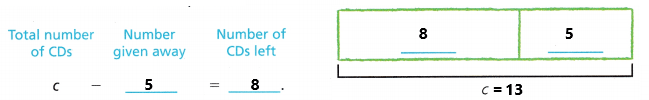Equation: c – 5 = 8
Add: c = 8 + 5 = 13
So, Kent had 13 CDs before he gave some away.
Explanation:
Kent has a collection of CDs. He gives 5 CDs to his brother. He then has 8 CDs left in his collection. First write the equation c – 5 = 8. Where c is the number of CDs in Kent’s collection before he gave some away. By using above strip diagram we can write the related addition equation. The addition equation is c = 8 + 5. Add 8 with 5 the sum is 13. So, Kent had 13 CDs before he gave some away.

Share and Show

Use the strip diagram to write an equation. Then solve.

Question 1.
Janine went to the beach 22 times during July and August. Six of those times were in August. how many times did she go in July?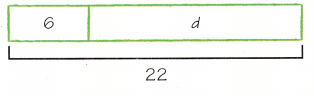Equation: ____________ ________ = d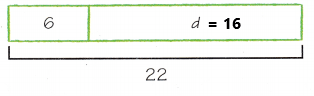Equation:
6 + d = 22
d = 22 – 6
d = 16
Janine went to the beach 16 times in July.
Explanation:
Janine went to the beach 22 times during July and August. Six of those times were in August. The equation is
6 + d = 22. Perform subtraction operation to find d value. Subtract 6 from 22 the difference is 16. Janine went to the beach 16 times in July.

Question 2.
Ariana has some crayons and her friend has 7 fewer crayons. If her friend has 15 crayons, how many crayons, c, does Ariana have?Equation: _____________ c = _________Equation:
c – 7 = 15
c = 15 + 7
c = 22
Ariana have 22 crayons.
Explanation:
Ariana has some crayons and her friend has 7 fewer crayons. Which means Ariana have more crayons than her friend. If her friend has 15 crayons then we have to calculate the Ariana crayons. The equation is c – 7 = 15. Perform addition operation to calculate the c value. Add 15 with 7 the sum is 22. So, Ariana have 22 crayons.

Question 3.
Explain how a strip diagram helps you understand the problem and write an equation.
In the above problem strip diagram is used to understand the problem and helped to writ the equation. First Ariana friend have 15 crayons. Ariana has some crayons and her friend has 7 fewer crayons. Which means 7 crayons are less than Ariana. So the equation is c – 7 = 15. Where c is Ariana’s crayons. Add 15 with 7 the sum is 22. So, Ariana have 22 crayons.

Problem Solving.

Use the bar graph to solve 4-6. Draw a strip diagram to write each equation. Then solve.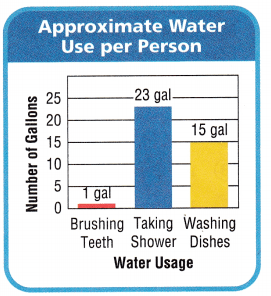Question 4.
H.O.T. Multi-Step Taking a shower uses about 13 fewer gallons of water than taking a bath. About how many gallons of water are used for taking a bath?
In your equation, let b represent the number of gallons of water needed for a bath.

Question 5.
H.O.T. Multi-Step Stephan did some chores before heading to practice. He washed the dishes and washed a load of laundry. He used about 44 gallons of water in all. How many gallons of water did he use to wash a load of laundry?Question 6.
H.O.T. Multi-Step You use 2 fewer gallons of water to brush your teeth than to wash your hands. How much water do you use to wash your hands?
In your equation, let h represent the number of gallons of water used to wash your hands.

Question 7.
Apply Wes had some money in his wallet and $39 in his pocket. He had$76 in all. He could represent his money by using the equation 39 + m = 76. How much did Wes have in his wallet?
$39 + m =$76
m = $76 –$39
m = $37 Wes have$37 in his wallet.
Explanation:
Wes had some money in his wallet and $39 in his pocket. He had$76 in all. He represented his money by using the equation 39 + m = 76. Perform subtraction operation. Subtract 39 from 76 the difference is $37. Wes have$37 in his wallet.

Question 8.
Stan spent $18 on giant carrots at the Giant Vegetable Store. He also bought a bag of giant peas, but the bag had no price tag. Stan was charged a total of$23. Which equation can help you find the cost of the peas?
(A) 18 + 23 = p
(B) 18 – 23 = p
(C) 18 + p = 23
(D) 23 + p = 18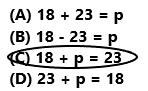The equation 18 + p = 23 is used to find the cost of the peas.
So, option C is correct.
Explanation:
Stan spent $18 on giant carrots at the Giant Vegetable Store. He also bought a bag of giant peas, but the bag had no price tag. Stan was charged a total of$23. So, the equation 18 + p = 23 is used to find the cost of the peas.
So, option C is correct.

Question 9.
Representations Morgan has 28 pieces of jewelry, 10 of which are necklaces. The rest of the jewelry pieces are bracelets. Which equation can you use to find the number of bracelets, b, she has?
(A) b = 10 – 28
(B) b = 28 – 10
(C) 28 = b – 10
(D) 10 = b + 28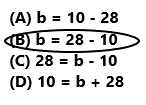The equation is 10 + b = 28
The equation b = 28 – 10 is used to find the number of bracelets.
Morgan has 18 bracelets. So, option B is correct.
Explanation:
Morgan has 28 pieces of jewelry, 10 of which are necklaces. The rest of the jewelry pieces are bracelets. The equation b = 28 – 10 is used to find the number of bracelets. Morgan has 18 bracelets. So, option B is correct.

Question 10.
Multi-Step Joseph bought 5 folders for school that cost $3 each. He also bought a new backpack. If the total cost was$35, how much did the backpack cost?
(A) $5 (B)$15
(C) $20 (D)$50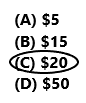5 x $3 =$15
The folders cost is $15.$15 + b = $35 b =$35 – $15 b =$20
The backpack cost is $20. So, option C is correct. Explanation: Joseph bought 5 folders for school that cost$3 each. He also bought a new backpack. The total cost was $35. First we have to calculate the folders cost. Multiply 5 folders with$3 the product is $15. The equation to calculate the new backpack cost is$15 + b = $35. Subtract$15 from $35 the difference is$20. The backpack cost is $20. So, option C is correct. Texas Test Prep Question 11. A football team scored 16 points, which was 12 fewer points than their opponents scored. How many points, y, did their opponents score? (A) y = 24 (B) y = 28 (C) y = 4 (D) y = 18 Answer:y – 16 = 12 y = 12 + 16 y = 28 Their opponents scored 28 points. SO, option B is correct. Explanation: A football team scored 16 points, which was 12 fewer points than their opponents scored. The equation y – 16 = 12 is used to calculate the opponents score. Perform addition operation. Add 12 with 16 the sum is 28. Their opponents scored 28 points. SO, option B is correct. ### Texas Go Math Grade 5 Lesson 8.2 Homework and Practice Answer Key Use the strip diagram to write an equation. Then solve. Question 1. Kiki had 17 mystery books. She got some more mystery books as a gift. Now she has a total of 21 mystery books. How many books did Kiki receive as a gift?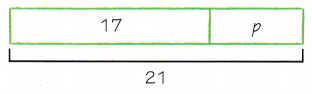Equation: _____________ _______ = p Answer:Equation: 17 + p = 21 p = 21 – 17 p = 4 Kiki receive 4 books as a gift. Explanation: Kiki had 17 mystery books. She got some more mystery books as a gift. Now she has a total of 21 mystery books. The equation is 17 + p = 21. Perform subtraction operation to calculate the p value. Subtract 17 books from 21 books the difference is 4 books. Kiki receive 4 books as a gift. Question 2. Joe scored 45 points. He scored 12 fewer points than Randy. How points did Randy score?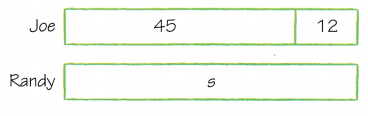Equation: _____________ s = _____________ Answer: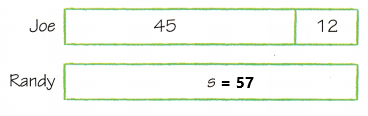Equation: s – 12 = 45 s = 45 + 12 s = 57 Randy scored 57 points. Explanation: Joe scored 45 points. He scored 12 fewer points than Randy. The equation is s – 12 = 45. To calculate the s value we need to perform addition operation. Add 45with 12 the sum is 57. So, Randy scored 57 points. Question 3. The pet store had some goldfish. Eight goldfish were sold. There are 28 goldfish left. How many goldfish did the pet store have before some were sold?Equation: ______________ f = ___________ Answer: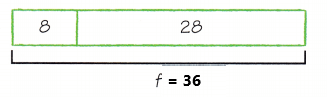8 + 28 = f 36 = f The pet store have 36 goldfish before some were sold. Explanation: The pet store had some goldfish. Eight goldfish were sold. There are 28 goldfish left. The equation is 8 + 28 = f. To calculate the f value we need to perform addition operation. Add 8 gold fish with 28 goldfish the sum is 36 goldfish. The pet store have 36 goldfish before some were sold. Question 4. Joaquin and Erik worked together on a project. Erik worked 13 hours. Together, they worked 22 hours. How many hours did Joaquin work?Equation: ____________ _______ = h Answer: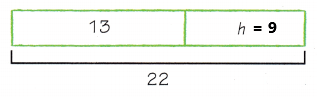Equation: 13 + h = 22 h = 22 – 13 h = 9 Joaquin worked 9 hours. Explanation: Joaquin and Erik worked together on a project. Erik worked 13 hours. Together, they worked 22 hours. The equation is 13 + h = 22. Perform subtraction operation to calculate the h value. Subtract 13 hours from 22 hours the difference is 9 hours. Joaquin worked 9 hours. Problem Solving Question 5. Nina arranges a bouquet of flowers for herself and for her mother. She uses 9 more daisies in her bouquet than in her mother’s bouquet. Her mother’s bouquet has 15 daisies. She represents the number of daisies in her bouquet by using the equation d – 9 = 15. How many daisies does Nina’s bouquet have? Answer: Equation: d – 9 = 15 d = 15 + 9 d = 24 Nina’s bouquet have 24 daisies. Explanation: Nina arranges a bouquet of flowers for herself and for her mother. She uses 9 more daisies in her bouquet than in her mother’s bouquet. Her mother’s bouquet has 15 daisies. She represents the number of daisies in her bouquet by using the equation d – 9 = 15. To calculate the d value we have to perform addition operation. Add 15 with 9 the sum is 24. Nina’s bouquet have 24 daisies. Question 6. Kierra has 49 pennies in a drawer and some pennies in a jar. She has 81 pennies in all. She represents the number of pennies by using the equation 49 + j = 81. How many pennies does Kierra have in the jar? Answer: Equation: 49 + j = 81 j = 81 – 49 j = 32 Kierra have 32 pennies in the jar. Explanation: Kierra has 49 pennies in a drawer and some pennies in a jar. She has 81 pennies in all. She represents the number of pennies by using the equation 49 + j = 81. Perform subtraction operation to calculate the j value. Subtract 49 from 81 the difference is 32. Kierra have 32 pennies in the jar. Lesson Check Fill in the bubble completely to show your answer. Question 7. Last weekend, Ryan earned$27 babysitting. He earned $19 on Friday and the rest of the money on Saturday. Which equation can you use to find the amount, m, Ryan earned on Saturday? (A) m =$27 + $19 (B)$19 = m + $27 (C)$27 = m – $19 (D) m =$27 – $19 Answer: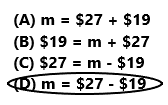Equation:$19 + m = $27 m =$27 – $19 m =$8
Ryan earned $8 on Saturday. So, option D is correct. Explanation: Last weekend, Ryan earned$27 babysitting. He earned $19 on Friday and the rest of the money on Saturday. The equation is m =$27 – $19. To calculate the m value we need to perform subtraction operation. Subtract$19 from $27 the difference is$8. Ryan earned $8 on Saturday. So, option D is correct. Question 8. Fatima used a coupon to pay for a pair of jeans. The original price of the jeans was$25. The coupon reduced the price to $16. Which equation can you use to help you find the value of the coupon? (A)$16 + c = $25 (B)$25 + $16 = c (C)$25 + c = $16 (D)$16 – c = $25 Answer:The equation$25 + $16 = c is used to find the value of the coupon. So, option B is correct. Explanation: Fatima used a coupon to pay for a pair of jeans. The original price of the jeans was$25. The coupon reduced the price to $16. The equation$25 + $16 = c is used to find the value of the coupon. So, option B is correct. Question 9. Hailey received 33 text messages on Saturday, which were 18 fewer messages than she received on Sunday. How many text messages, t, did Hailey receive on Sunday? (A) t = 15 (B) t = 25 (C) t = 51 (D) t = 41 Answer: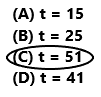t – 18 = 33 t = 33 + 18 t = 51 Hailey received 51 text messages on Sunday. So, option C is correct. Explanation: Hailey received 33 text messages on Saturday, which were 18 fewer messages than she received on Sunday. The equation is t – 18 = 23. To calculate the t value we have to perform addition operation. Add 33 with 18 the sum is 51. Hailey received 51 text messages on Sunday. So, option C is correct. Question 10. At the second bus stop, 26 people got off the bus, which were 9 more than the number of people that got off at the first bus stop. How many people, p, got off the bus at the first stop? (A) P = 17 (B) p = 35 (C) P = 23 (D) p = 15 Answer: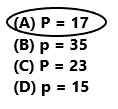p + 9 = 26 p = 26 – 9 p = 17 At the first bus stop 17 people got off the bus. So, option A is correct. Explanation: At the second bus stop, 26 people got off the bus, which were 9 more than the number of people that got off at the first bus stop. The equation is p + 9 = 26. To calculate the p value we have to perform subtraction operation. Subtract 9 people from 26 people the difference is 17 people. At the first bus stop 17 people got off the bus. So, option A is correct. Question 11. Multi-Step There were 14 dogs adopted from the animal shelter last week. This week, 8 dogs were adopted. There are 5 dogs left. How many dogs, d, did the shelter have for adoption? (A) d = 27 (B) d = 22 (C) d = 13 (D) d = 17 Answer:14 + 8 = 22 22 – 5 = 17 17 dogs have the shelter for adoption. So, option D is correct. Explanation: There were 14 dogs adopted from the animal shelter last week. This week, 8 dogs were adopted. Add 14 dogs with 8 dogs the sum is 22 dogs. There are 5 dogs left. Subtract 5 dogs from 22 dogs the difference is 17 dogs. 17 dogs have the shelter for adoption. So, option D is correct. Question 12. Multi-Step Lorenzo spent$47 at the shoe store. He bought 3 pairs of socks that cost $6 per pair. He also bought a pair of shoes. He paid$4 in sales tax. How much did Lorenzo’s
shoes, s, cost?
(A) s = $25 (B) s =$22
(C) s = $34 (D) s =$29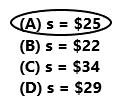He bought 3 pairs of socks that cost $6 per pair. 3 x$6 = $18 He paid$4 in sales tax.
$18 +$4 = $22 Lorenzo spent$47 at the shoe store.
$47 –$22 = $25 Lorenzo’s shoes cost is$25.
Lorenzo spent $47 at the shoe store. He bought 3 pairs of socks that cost$6 per pair. Multiply 3 with $6the product is$18. The 3 pairs of socks cost is $18. He also bought a pair of shoes. He paid$4 in sales tax. Add $18 with$4 the sum is $22. Subtract$22 from $47 the difference is$25. Lorenzo’s shoes cost is \$25. So, option A is correct.